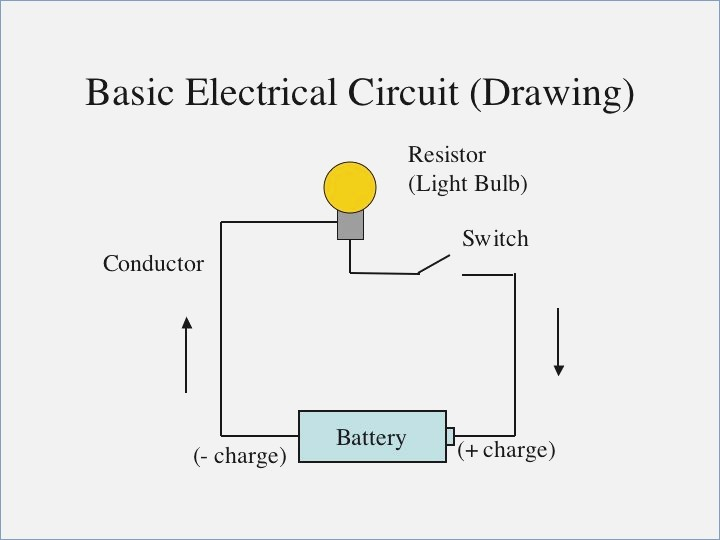Angel Pinto

# CBSE Class 10 Science Chapter 12 Revision Notes

An electric circuit is a closed-loop channel that a current follows. A circuit diagram is a visual representation of an electric circuit using symbols. Friction brought the notion of electricity to light.

Table of content

# Chapter 12: Electricity Revision Notes

Structure of the Atom

• The nucleus of an atom is positively charged, while the electrons that orbit it are negatively charged.
• Metals' valence electrons are free to move around within the conductor, creating an electric current.

Charge

• Charge is a fundamental feature of matter that allows it to exert electromagnetic force.

Insulators and Conductors

• Conductors are substances that provide comparatively less resistance to the passage of electricity, whereas insulators provide more resistance.

Potential Difference and Electric Potential

• The effort done to move a unit positive charge from infinity to that location is defined as the electric potential at that place.
• The difference in electric potentials between two points is defined as the potential difference between two points.

## MODELS OF ELECTRIC CURRENT

Electric Current(I)

• Electric current (I = Q / t) is the flow of electric charges.

Electron sea model

• The drift of a 'sea' of free electrons, which are free to leap onto any nearby atom, causes electric current in a solid conductor.

Drift velocity of Electron

• The average velocity that an electron achieves inside a metallic conductor when an electric field is applied owing to a potential difference.

Battery and its working

• A cell is a source of potential difference that is generated internally by chemical processes.
• At anode: Cu(s)⇌Cu2+(aq)+2e−
• At cathode: Ag(aq)+2e−⇌2Ag(s)
• The term "battery" refers to a collection of cells.

Electric CircuitSource:

• An electric circuit is a closed-loop channel that a current follows.
• A circuit diagram is a visual representation of an electric circuit using symbols.

## OHM’S LAW AND RESISTANCE

Ohm’s law

• The applied potential difference between the two ends of an ohmic conductor is precisely proportional to the current flowing through it.

Resistance

• A measure of the resistance to current flow in an electric circuit is called resistance.

Factors affecting Resistance & How they affect

The resistance of a conductor :

• is proportional to its length.
• directly proportionate to the conductor's nature
• directly proportional to the temperature of the conductor.
• inversely proportional to the conductor's cross-sectional area

Resistivity

• Resistivity is the electrical resistance provided by a material of unit length and cross-sectional area.

Ohmic and Non-Ohmic resistors

• Ohmic resistors are those that follow Ohm's Law, whereas Non-Ohmic resistors are those that do not.

Superconductors

• Superconductors are conductors that have no resistance to the passage of electricity.

Combination of Resistors

• If two resistors carry the same current, they are said to be in series.
• When the same potential difference is applied to two resistors, they are said to be connected in parallel.

The equivalent resistance of two resistors can be calculated as follows:

In series, Req=R1+R2

In parallel, 1/Req= 1/R1+ 1/R2

Terminal Voltage and EMF

• When there is no current flowing through the circuit, the potential difference between the two terminals of a cell is called EMF.
• When current is flowing through a circuit, the potential difference between the two terminals of a cell is called terminal voltage. ​​

## Heating Effect of Current

Joule’s Law:

• Heat (H) ∝ square of the current (I).
• H ∝ Resistance of the given circuit.
• H ∝ Time (t) for which current flows through the conductor.
• When a potential difference is generated, electrons travel, resulting in current flow.

Electric Power

• Electric power is the rate at which work is completed or electrical energy is used. If W is the amount of work completed in time t, then P=W/t.
• Watt is the SI unit (W). When 1 A of current flows at a potential difference of 1 V, one watt of electricity is consumed.
• A kilowatt-hour is a commercial unit of electrical energy (kWh).
• 3.6106J = 1 kWh = 3,600,000J
• P=I2R and P=V2/R are two symbols for the same thing.
• The amount of energy consumed when 1kW of electricity is used for 1 hour is defined as one kilowatt-hour.

Source: